# How I rediscovered Logo with the Python Turtle module

## The Logo programming language is available today as a Python package.Image credits :

Photo by Patti Black on Unsplash

## Subscribe now

Get the highlights in your inbox every week.

When I was in high school, one of the very first programming languages I was introduced to was Logo. It was interactive and visual. With basic movement commands, you could have your cursor (“turtle”) draw basic shapes and intricate patterns. It was a great way to introduce the compelling concept of an algorithm—a series of instructions for a computer to execute.

Fortunately, the Logo programming language is available today as a Python package. So let’s jump right in, and you can discover the possibilities with Logo as we go along.

## Installing the Turtle module

Logo is available as the `turtle` package for Python. To use it, you must have Python installed first. Python is already installed on Linux and BSD, and it's easy to install on both MacOS and Windows.

Once you have Python installed, install the Turtle module:

``pip3 install turtle``

## Bob draws a square

With the `turtle` package installed, you can draw some basic shapes.

To draw a square, imagine a turtle (call him Bob) in the middle of your screen, holding a pen with his tail. Every time Bob moves, he draws a line behind him. How must Bob move to draw a square?

1. Move forward 100 steps.
2. Turn right 90 degrees.
3. Move forward 100 steps.
4. Turn right 90 degrees.
5. Move forward 100 steps.
6. Turn right 90 degrees.
7. Move forward 100 steps.

Now write the above algorithm in Python. Create a file called `logo.py` and place the following code in it.

```import turtle

if __name__ == '__main__':

turtle.title('Hi! I\'m Bob the turtle!')
turtle.setup(width=800, height=800)

bob = turtle.Turtle(shape='turtle')
bob.color('orange')

# Drawing a square
bob.forward(100)
bob.right(90)
bob.forward(100)
bob.right(90)
bob.forward(100)
bob.right(90)
bob.forward(100)

turtle.exitonclick()```

Save the above as `logo.py` and run it:

``\$ python3 logo.py``

Bob draws a square on the screen:

## rediscovering-logo-python-turtle-square.jpg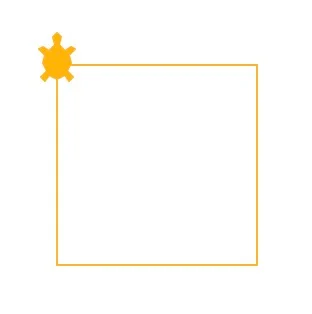Illustration by Ayush Sharma, CC BY-SA 4.0

## Bob draws a hexagon

To draw a hexagon, Bob must move like this:

1. Move forward 150 steps.
2. Turn right 60 degrees.
3. Move forward 150 steps.
4. Turn right 60 degrees.
5. Move forward 150 steps.
6. Turn right 60 degrees.
7. Move forward 150 steps.
8. Turn right 60 degrees.
9. Move forward 150 steps.
10. Turn right 60 degrees.
11. Move forward 150 steps.

In Python, you can use a `for` loop to move Bob:

```import turtle

if __name__ == '__main__':

turtle.title('Hi! I\'m Bob the turtle!')
turtle.setup(width=800, height=800)

bob = turtle.Turtle(shape='turtle')
bob.color('orange')

# Drawing a hexagon
for i in range(6):

bob.forward(150)
bob.right(60)

turtle.exitonclick()```

Run your code again and watch Bob draw a hexagon.

## rediscovering-logo-python-turtle-hexagon.jpg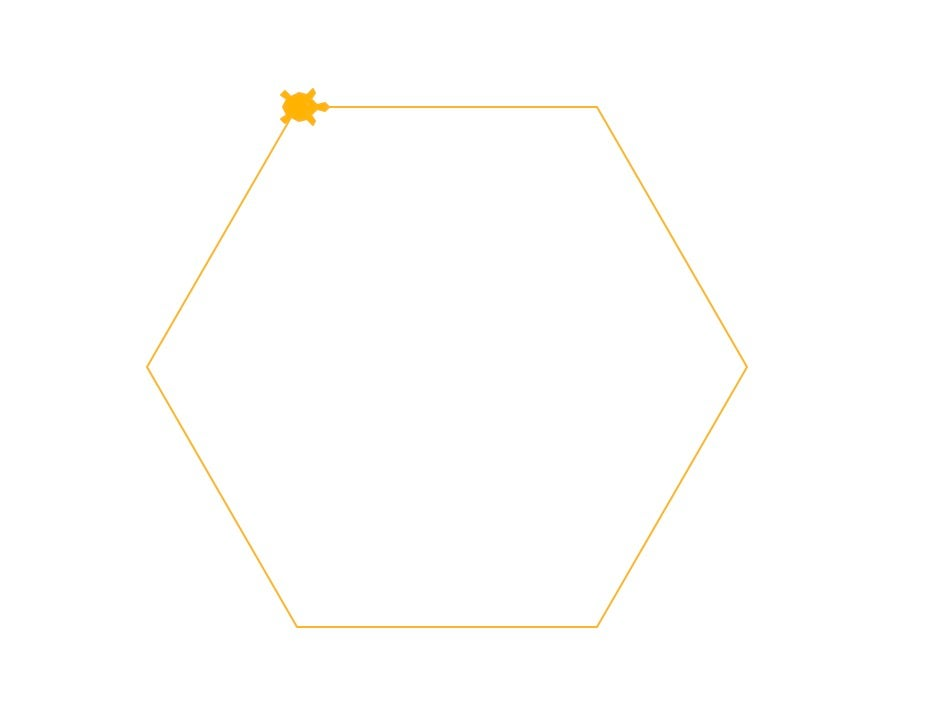Illustration by Ayush Sharma, CC BY-SA 4.0

## Bob draws a square spiral

Now try drawing a square spiral, but this time you can speed things up a bit. You can use the `speed` function and set `bob.speed(2000)` so that Bob moves faster.

```import turtle

if __name__ == '__main__':

turtle.title('Hi! I\'m Bob the turtle!')
turtle.setup(width=800, height=800)

bob = turtle.Turtle(shape='turtle')
bob.color('orange')

# Drawing a square spiral
bob.speed(2000)
for i in range(500):

bob.forward(i)
bob.left(91)

turtle.exitonclick()```

## rediscovering-logo-python-turtle-square-spiral.jpg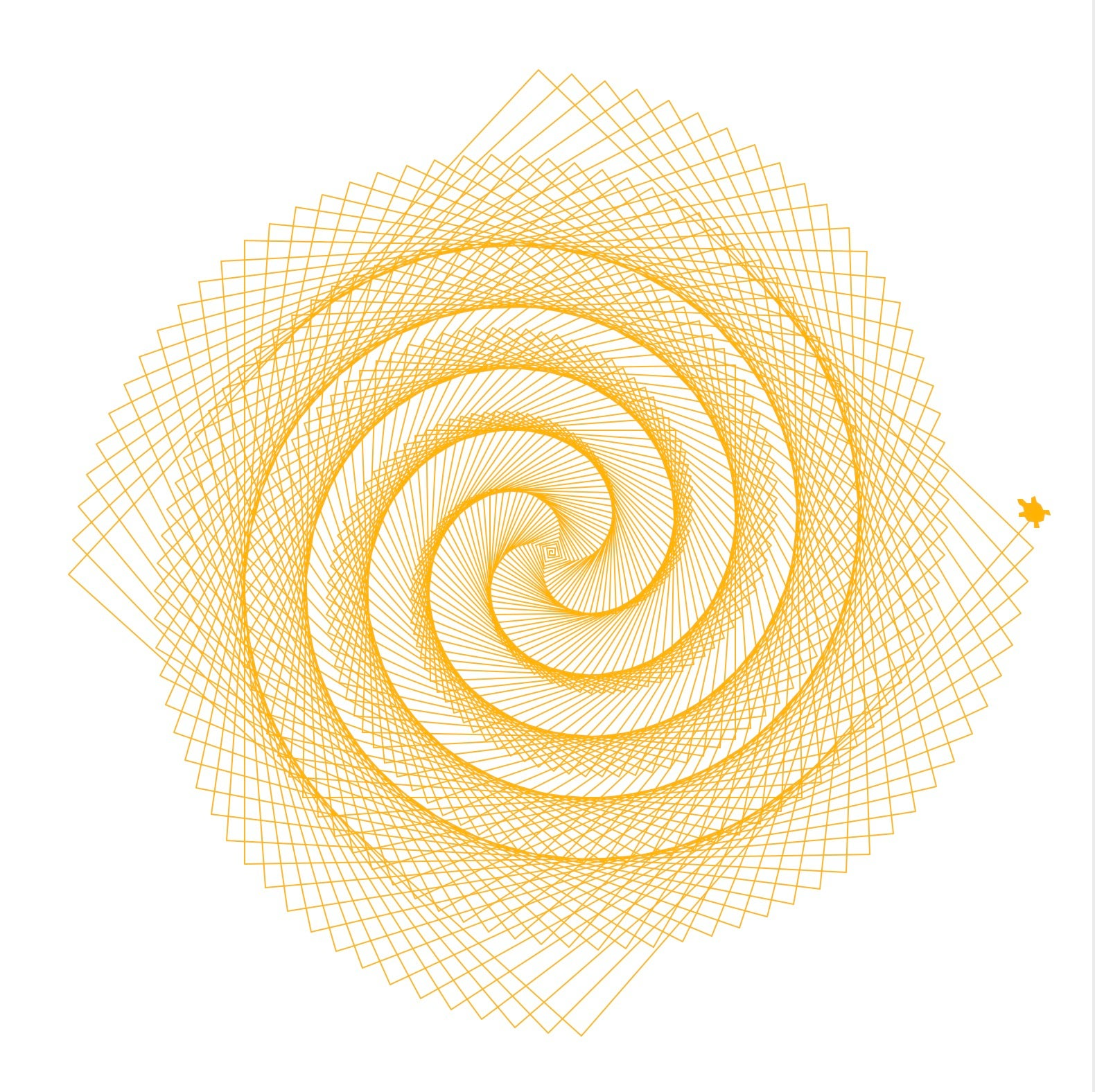Illustration by Ayush Sharma, CC BY-SA 4.0

## Bob and Larry draw a weird snake thing

In the above examples, you initialized `Bob` as an object of the `Turtle` class. You're not limited to just one turtle, though. In the next code block, create a second turtle called `Larry` to draw along with Bob.

The `penup()` function makes the turtles lift their pens, so they don’t draw anything as they move, and the `stamp()` function places a marker whenever it’s called.

```import turtle

if __name__ == '__main__':

turtle.title('Hi! We\'re Bob and Larry!')
turtle.setup(width=800, height=800)

bob = turtle.Turtle(shape='turtle')
larry = turtle.Turtle(shape='turtle')
bob.color('orange')
larry.color('purple')

bob.penup()
larry.penup()
bob.goto(-180, 200)
larry.goto(-150, 200)
for i in range(30, -30, -1):

bob.stamp()
larry.stamp()
bob.right(i)
larry.right(i)
bob.forward(20)
larry.forward(20)

turtle.exitonclick()```

## rediscovering-logo-python-turtle-stamping-larry.jpg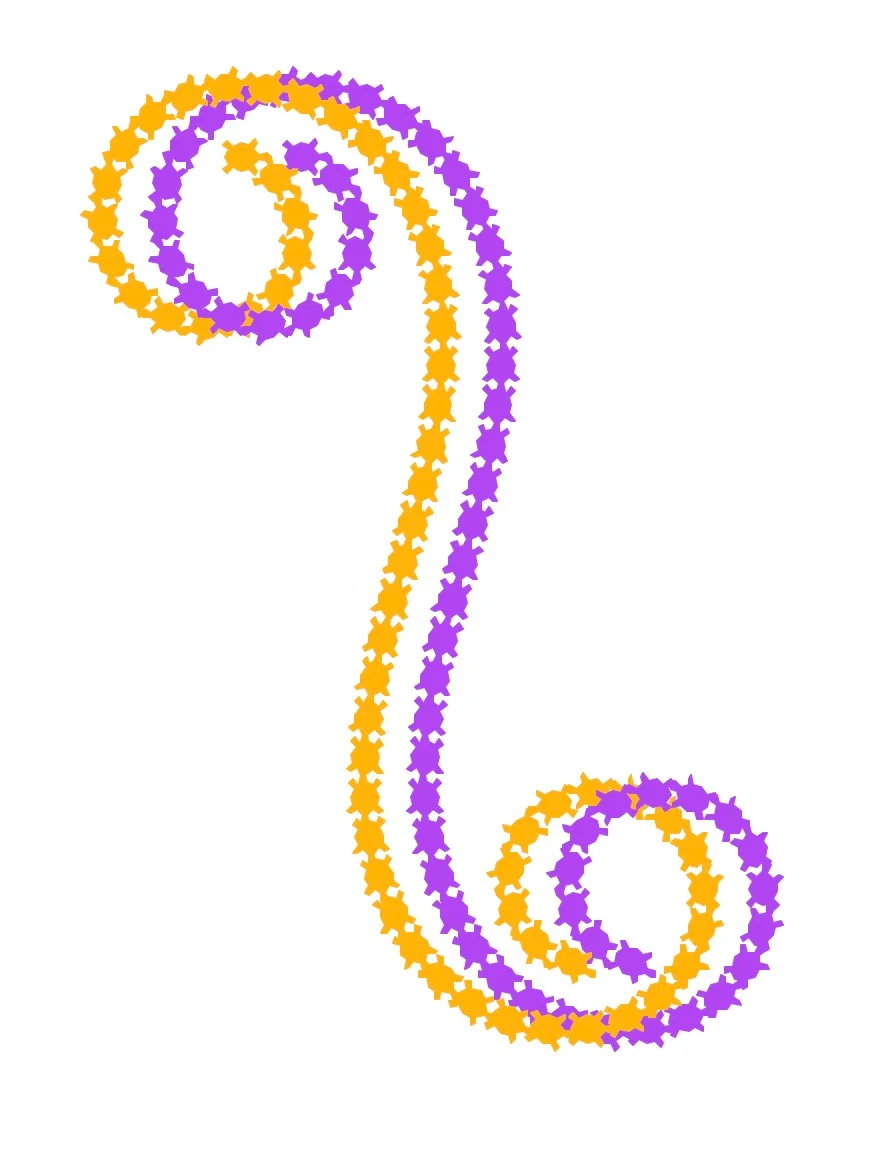Illustration by Ayush Sharma, CC BY-SA 4.0

## Bob draws a sunburst

Bob can also draw simple lines and fill them in with color. The functions `begin_fill()` and `end_fill()` allow Bob to fill a shape with the color set with `fillcolor()`.

```import turtle

if __name__ == '__main__':

turtle.title('Hi! I\'m Bob the turtle!')
turtle.setup(width=800, height=800)

bob = turtle.Turtle(shape='turtle')
bob.color('orange')

# Drawing a filled star thingy
bob.speed(2000)
bob.fillcolor('yellow')
bob.pencolor('red')

for i in range(200):

bob.begin_fill()
bob.forward(300 - i)
bob.left(170)
bob.forward(300 - i)
bob.end_fill()

turtle.exitonclick()```

## rediscovering-logo-python-turtle-sunburst.jpg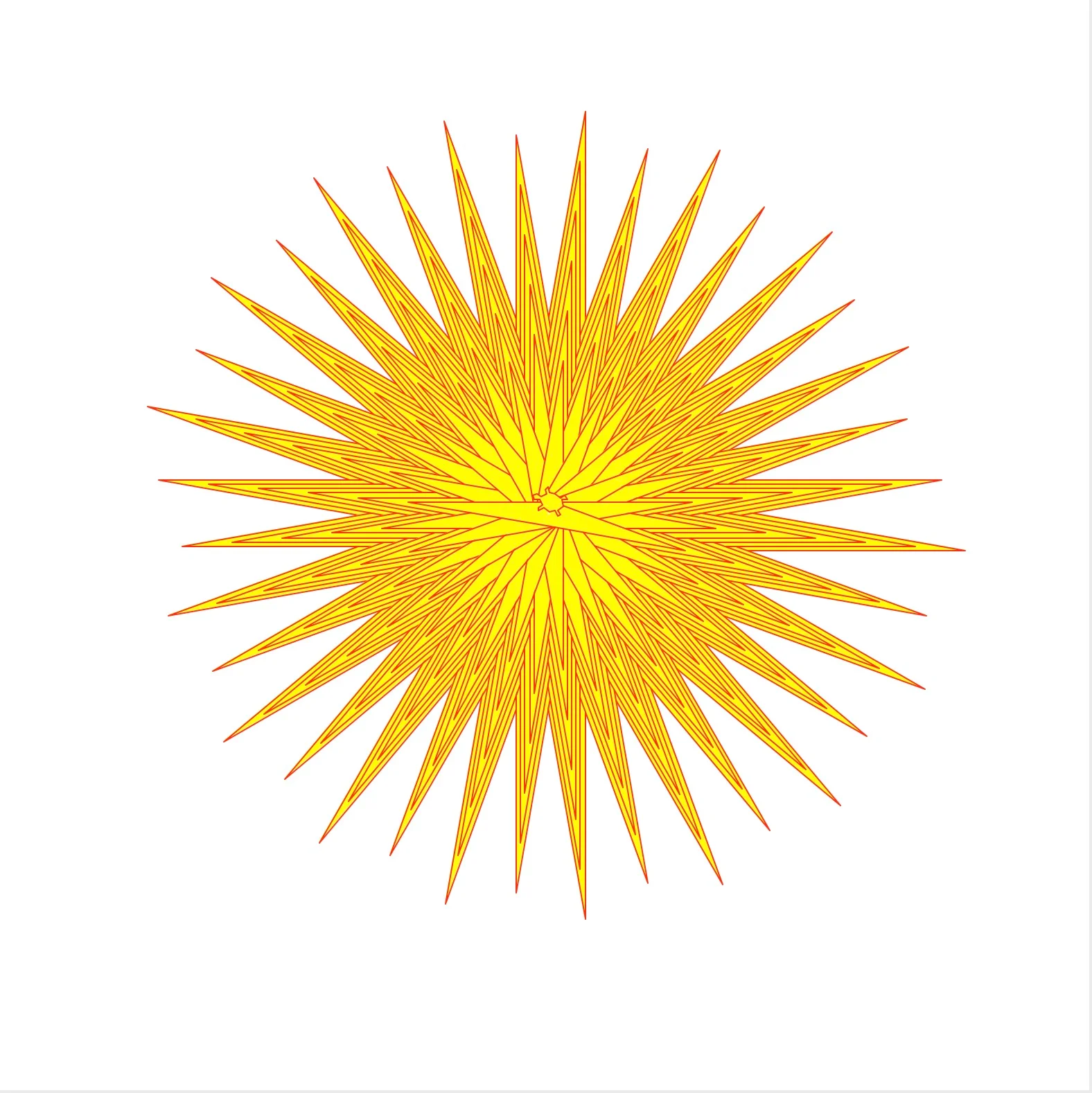Illustration by Ayush Sharma, CC BY-SA 4.0

## Larry draws a Sierpinski triangle

Bob enjoys drawing simple geometrical shapes holding a pen with his tail as much as the next turtle, but what he enjoys most is drawing fractals.

One such shape is the Sierpinski triangle, which is an equilateral triangle recursively subdivided into smaller equilateral triangles. It looks something like this:

## rediscovering-logo-python-turtle-sierpinski-triangle.jpg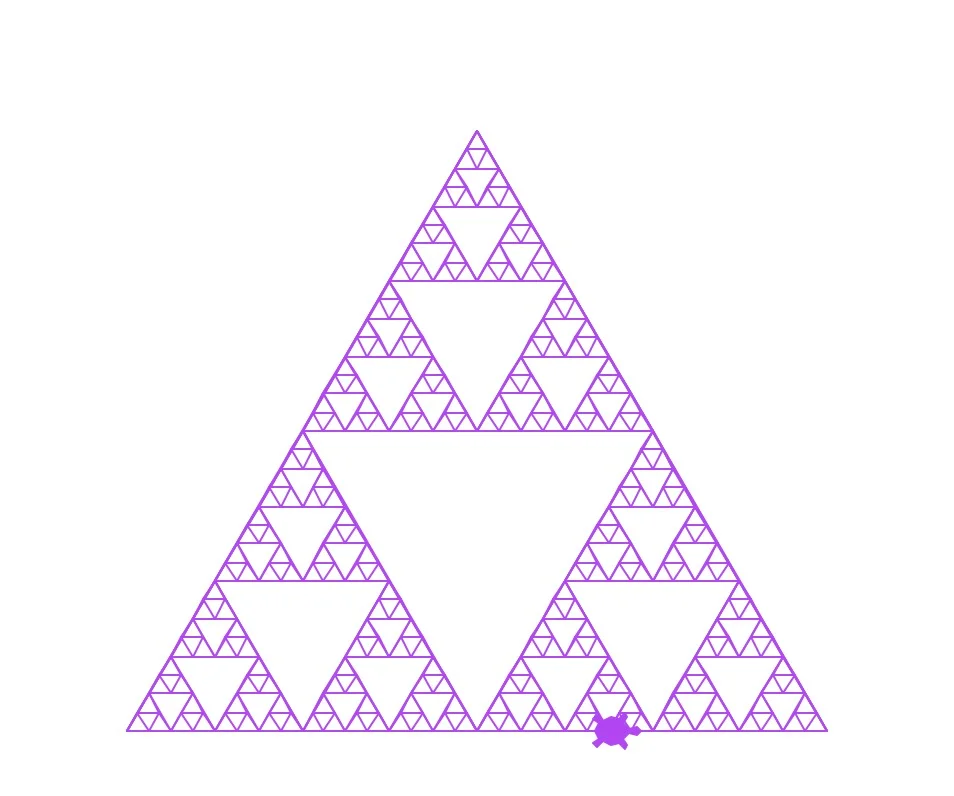Illustration by Ayush Sharma, CC BY-SA 4.0

To draw the Sierpinski triangle above, Bob has to work a bit harder:

```import turtle

def get_mid_point(point_1: list, point_2: list):

return ((point_1 + point_2) / 2, (point_1 + point_2) / 2)

def triangle(turtle: turtle, points, depth):

turtle.penup()
turtle.goto(points, points)

turtle.pendown()
turtle.goto(points, points)
turtle.goto(points, points)
turtle.goto(points, points)

if depth > 0:

triangle(turtle, [points, get_mid_point(points, points), get_mid_point(points, points)], depth-1)
triangle(turtle, [points, get_mid_point(points, points), get_mid_point(points, points)], depth-1)
triangle(turtle, [points, get_mid_point(points, points), get_mid_point(points, points)], depth-1)

if __name__ == '__main__':

turtle.title('Hi! I\'m Bob the turtle!')
turtle.setup(width=800, height=800)

larry = turtle.Turtle(shape='turtle')
larry.color('purple')

points = [[-175, -125], [0, 175], [175, -125]]  # size of triangle

triangle(larry, points, 5)

turtle.exitonclick()```

## Wrap up

The Logo programming language is a great way to teach basic programming concepts, such as how a computer can execute a set of commands. Also, because the library is now available in Python, it can be used to visualize complex ideas and concepts.

I hope Bob and Larry have been enjoyable and instructive.

Have fun, and happy coding.